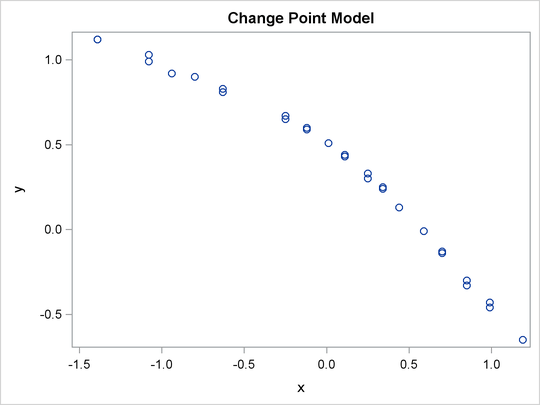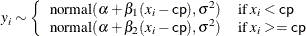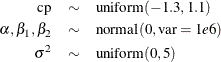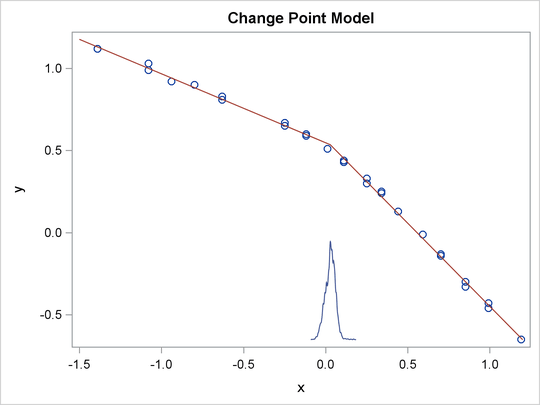# The MCMC Procedure

### Example 61.12 Change Point Models

Consider the data set from Bacon and Watts (1971), whereis the logarithm of the height of the stagnant surface layer and the covariateis the logarithm of the flow rate of water. The following statements create the data set:

title 'Change Point Model';
data stagnant;
input y x @@;
ind = _n_;
datalines;
1.12  -1.39   1.12  -1.39   0.99  -1.08   1.03  -1.08
0.92  -0.94   0.90  -0.80   0.81  -0.63   0.83  -0.63
0.65  -0.25   0.67  -0.25   0.60  -0.12   0.59  -0.12
0.51   0.01   0.44   0.11   0.43   0.11   0.43   0.11
0.33   0.25   0.30   0.25   0.25   0.34   0.24   0.34
0.13   0.44  -0.01   0.59  -0.13   0.70  -0.14   0.70
-0.30   0.85  -0.33   0.85  -0.46   0.99  -0.43   0.99
-0.65   1.19
;


A scatter plot (Output 61.12.1) shows the presence of a nonconstant slope in the data. This suggests a change point regression model (Carlin, Gelfand, and Smith, 1992). The following statements generate the scatter plot in Output 61.12.1:

ods graphics on;
proc sgplot data=stagnant;
scatter x=x y=y;
run;


Output 61.12.1: Scatter Plot of the Stagnant Data SetLet the change point be cp. Following formulation by Spiegelhalter et al. (1996b), the regression model is as follows:You might consider the following diffuse prior distributions:The following statements generate Output 61.12.2:

proc mcmc data=stagnant outpost=postout seed=24860 ntu=1000
nmc=20000;
ods select PostSumInt;
ods output PostSumInt=ds;

array beta;
parms alpha cp beta1 beta2;
parms s2;

prior cp ~ unif(-1.3, 1.1);
prior s2  ~ uniform(0, 5);
prior alpha beta:  ~ normal(0, v = 1e6);

j = 1 + (x >= cp);
mu = alpha + beta[j] * (x - cp);
model y ~ normal(mu, var=s2);
run;


The PROC MCMC statement specifies the input data set (Stagnant), the output data set (Postout), a random number seed, a tuning sample of 1000, and an MCMC sample of 20000. The ODS SELECT statement displays only the summary statistics table. The ODS OUTPUT statement saves the summary statistics table to the data set Ds.

The ARRAY statement allocates an array of size 2 for the beta parameters. You can use beta1 and beta2 as parameter names without allocating an array, but having the array makes it easier to construct the likelihood function. The two PARMS statements put the five model parameters in two blocks. The three PRIOR statements specify the prior distributions for these parameters.

The symbol j indicates the segment component of the regression. When x is less than the change point, (x >= cp) returns 0 and j is assigned the value 1; if x is greater than or equal to the change point, (x >= cp) returns 1 and j is 2. The symbol mu is the mean for the jth segment, and beta[j] changes between the two regression coefficients depending on the segment component. The MODEL statement assigns the normal model to the response variable y.

Posterior summary statistics are shown in Output 61.12.2.

Output 61.12.2: MCMC Estimates of the Change Point Regression Model

 Change Point Model

The MCMC Procedure

Posterior Summaries and Intervals
Parameter N Mean Standard
Deviation
95% HPD Interval
alpha 20000 0.5349 0.0249 0.4843 0.5813
cp 20000 0.0283 0.0314 -0.0353 0.0846
beta1 20000 -0.4200 0.0146 -0.4482 -0.3911
beta2 20000 -1.0136 0.0167 -1.0476 -0.9817
s2 20000 0.000451 0.000145 0.000220 0.000735

You can use PROC SGPLOT to visualize the model fit. Output 61.12.3 shows the fitted regression lines over the original data. In addition, on the bottom of the plot is the kernel density of the posterior marginal distribution of cp, the change point. The kernel density plot shows the relative variability of the posterior distribution on the data plot. You can use the following statements to create the plot:

data _null_;
set ds;
call symputx(parameter, mean);
run;

data b;
missing A;
input x1 @@;
if x1 eq .A then x1 = &cp;
if _n_ <= 2 then y1 = &alpha + &beta1 * (x1 - &cp);
else y1 = &alpha + &beta2 * (x1 - &cp);
datalines;
-1.5 A 1.2
;

proc kde data=postout;
univar cp / out=m1 (drop=count);
run;

data m1;
set m1;
density = (density / 25) - 0.653;
run;

data all;
set stagnant b m1;
run;

proc sgplot data=all noautolegend;
scatter x=x y=y;
series x=x1 y=y1 / lineattrs = graphdata2;
series x=value y=density / lineattrs = graphdata1;
run;
ods graphics off;


The macro variables &alpha, &beta1, &beta2, and &cp store the posterior mean estimates from the data set Ds. The data set b contains three predicted values, at the minimum and maximum values of x and the estimated change point &cp. These input values give you fitted values from the regression model. Data set M1 contains the kernel density estimates of the parameter cp. The density is scaled down so the curve would fit in the plot. Finally, you use PROC SGPLOT to overlay the scatter plot, regression line and kernel density plots in the same graph.

Output 61.12.3: Estimated Fit to the Stagnant Data Set